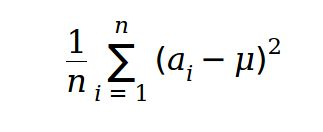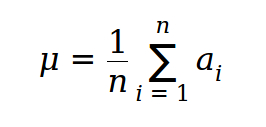# Uncle MSUB Inter University Prog...
Limits 2s, 512 MB

J's uncle Mr. M is obsessed with statistics. Wherever he finds data he likes to apply statistical analysis on that. J being his nephew M expects him to be good on statistical analysis as well. Today he's come to J with a 2D matrix of numbers from GOD knows where. Now he's choosing a rectangular portion from that matrix and asking J to calculate the variance of that portion. J is not that bad at statistics as well but needs your help to double check the answer before telling it to uncle M. Are you up for helping J?

For a set of numbers a1, a2, a3, … … … , an their variance is defined as ―where## Input

The first the of the input contains r(1≤r≤1000) and c(1≤c≤1000), the number of rows and the numbers of columns in the matrix. Then r lines follows with c numbers each. The number at i-th row and j-th column is aij(1≤aij≤10^5).
Then there is q(1≤q≤10^5) on a single line. Then follows q lines with four numbers x1, y1, x2, y2(1<=x1<=x2<=r, 1<=y1<=y2<=c) each where (x1, y1) is the upper left coordinate of the rectangle and (x2, y2) is the lower right coordinate of the rectangle.

## Output

For each query in the input output the variance of that rectangle on a single line. Errors less than 10-4 will be ignored.

## Sample

InputOutput
2 2
84 87
78 16
2
1 1 1 2
1 1 2 2

2.2500000000
852.1875000000


### Submit